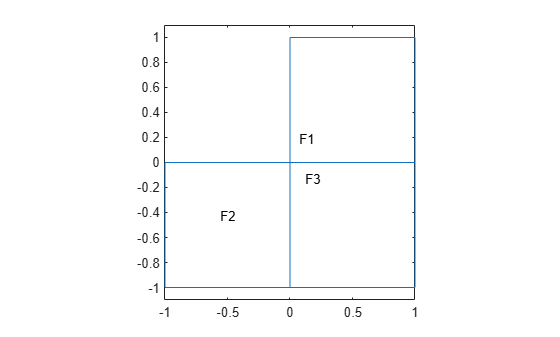# findThermalIC

Find thermal initial conditions assigned to a geometric region

## Syntax

``tica = findThermalIC(thermalmodel.InitialConditions,RegionType,RegionID)``

## Description

example

````tica = findThermalIC(thermalmodel.InitialConditions,RegionType,RegionID)` returns the thermal initial condition assigned to the specified region.```

## Examples

collapse all

Create a transient thermal model that has three faces.

```thermalmodel = createpde('thermal','transient'); geometryFromEdges(thermalmodel,@lshapeg); pdegplot(thermalmodel,'FaceLabels','on') ylim([-1.1 1.1]) axis equal```Set initial temperatures for each face.

```thermalIC(thermalmodel,10,'Face',1); thermalIC(thermalmodel,20,'Face',2); thermalIC(thermalmodel,30,'Face',3);```

Check the initial condition specification for face 1.

`ticaFace1 = findThermalIC(thermalmodel.InitialConditions,'Face',1)`
```ticaFace1 = GeometricThermalICs with properties: RegionType: 'face' RegionID: 1 InitialTemperature: 10 ```

Check the initial temperature specifications for faces 2 and 3.

```tica = findThermalIC(thermalmodel.InitialConditions,'Face',[2 3]); ticaFace2 = tica(1)```
```ticaFace2 = GeometricThermalICs with properties: RegionType: 'face' RegionID: 2 InitialTemperature: 20 ```
`ticaFace3 = tica(2)`
```ticaFace3 = GeometricThermalICs with properties: RegionType: 'face' RegionID: 3 InitialTemperature: 30 ```

Create a geometry that consists of three nested cylinders and include the geometry in a transient thermal model.

`gm = multicylinder([5 10 15],2)`
```gm = DiscreteGeometry with properties: NumCells: 3 NumFaces: 9 NumEdges: 6 NumVertices: 6 Vertices: [6x3 double] ```
```thermalmodel = createpde('thermal','transient'); thermalmodel.Geometry = gm; pdegplot(thermalmodel,'CellLabels','on','FaceAlpha',0.5)```Set initial temperatures for each cell.

```thermalIC(thermalmodel,0,'Cell',1); thermalIC(thermalmodel,100,'Cell',2); thermalIC(thermalmodel,0,'Cell',3);```

Check the initial condition specification for cell 1.

`ticaCell1 = findThermalIC(thermalmodel.InitialConditions,'Cell',1)`
```ticaCell1 = GeometricThermalICs with properties: RegionType: 'cell' RegionID: 1 InitialTemperature: 0 ```

Check the initial condition specification for cells 2 and 3.

```tica = findThermalIC(thermalmodel.InitialConditions,'Cell',[2:3]); ticaCell2 = tica(1)```
```ticaCell2 = GeometricThermalICs with properties: RegionType: 'cell' RegionID: 2 InitialTemperature: 100 ```
`ticaCell3 = tica(2)`
```ticaCell3 = GeometricThermalICs with properties: RegionType: 'cell' RegionID: 3 InitialTemperature: 0 ```

Create a thermal model and include a square geometry.

```thermalmodel = createpde('thermal','transient'); gm = @squareg; geometryFromEdges(thermalmodel,gm); pdegplot(thermalmodel,'FaceLabels','on') ylim([-1.1 1.1]) axis equal```Specify material properties, heat source, set initial and boundary conditions.

```thermalProperties(thermalmodel,'ThermalConductivity',500,... 'MassDensity',200,... 'SpecificHeat',100); internalHeatSource(thermalmodel,2); thermalBC(thermalmodel,'Edge',[1 3],'Temperature',100); thermalIC(thermalmodel,0);```

Generate a mesh and solve the problem.

```generateMesh(thermalmodel); tlist = 0:0.5:10; result1 = solve(thermalmodel,tlist)```
```result1 = TransientThermalResults with properties: Temperature: [1541x21 double] SolutionTimes: [0 0.5000 1 1.5000 2 2.5000 3 3.5000 4 4.5000 5 ... ] XGradients: [1541x21 double] YGradients: [1541x21 double] ZGradients: [] Mesh: [1x1 FEMesh] ```

Check the currently active initial temperature specification.

`tica = findThermalIC(thermalmodel.InitialConditions,'Face',1)`
```tica = GeometricThermalICs with properties: RegionType: 'face' RegionID: 1 InitialTemperature: 0 ```

Now, resume the analysis and solve the problem for times from 10 to 15 seconds. Use the previously obtained solution for 10 seconds as an initial condition. Since 10 seconds is the last element in `tlist`, you do not need to specify the solution time index. By default, `thermalIC` uses the last solution index.

`ic = thermalIC(thermalmodel,result1);`

Solve the problem

```tlist = 10:0.5:15; result2 = solve(thermalmodel,tlist);```

Check the currently active initial temperature specification.

`tica = findThermalIC(thermalmodel.InitialConditions,'Face',1)`
```tica = NodalThermalICs with properties: InitialTemperature: [1541x1 double] ```
`pdeplot(thermalmodel,'XYData',tica.InitialTemperature)`## Input Arguments

collapse all

Initial conditions of a thermal model, specified as the `InitialConditions` property of a `ThermalModel` object.

Geometric region type, specified as `'Edge'`, `'Face'`, or `'Vertex'` for a 2-D model or 3-D model, or `'Cell'` for a 3-D model.

Data Types: `char` | `string`

Geometric region ID, specified as a vector of positive integers. Find the region IDs using the `pdegplot` function with the `'FaceLabels'` (3-D) or `'EdgeLabels'` (2-D) value set to `'on'`.

Data Types: `double`

## Output Arguments

collapse all

Thermal initial condition for a particular region, returned as a GeometricThermalICs Properties or NodalThermalICs Properties object.